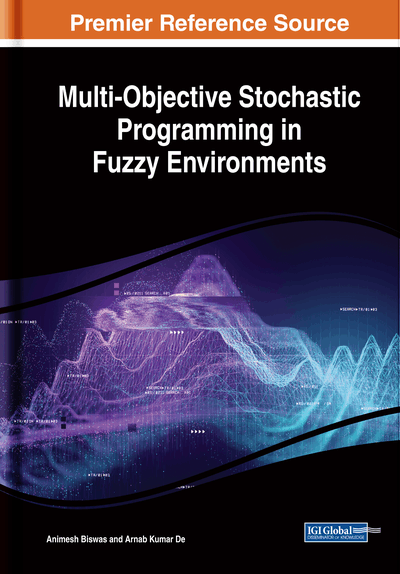# Development of Fuzzy Multi-Objective Stochastic Fractional Programming Models

DOI: 10.4018/978-1-5225-8301-1.ch004
Available
\$33.75
List Price: \$37.50
10% Discount:-\$3.75
TOTAL SAVINGS: \$3.75

## Abstract

In this chapter, two methodologies for solving multi-objective linear fractional stochastic programming problems containing fuzzy numbers (FNs) and fuzzy random variables (FRVs) associated with the system constraints are developed. In the model formulation process, the fuzzy probabilistic constraints are converted into equivalent fuzzy constraints by applying chance constrained programming (CCP) technique in a fuzzily defined probabilistic decision-making situation. Then two techniques, -cut and defuzzification methods, are used to convert the model into the corresponding deterministic model. In the method of using -cut for FNs, the tolerance level of FNs is considered, and the constraints are reduced to constraints with interval coefficients. Alternatively, in using defuzzification method, FNs are replaced by their defuzzified values. Consequently, the constraints are modified into constraints in deterministic form. In the next step, the constraints with interval coefficients are customized into its equivalent form by using the convex combination of each interval. If the parameters of the objectives are triangular FNs, then on the basis of their tolerance ranges each objective is decomposed into three objectives with crisp coefficients. Then each objective is solved independently to find their best and worst values and those values are used to construct membership function of each objective. Finally, the compromise solution of multi-objective linear fractional CCP problems is obtained by applying any of the approaches: priority-based fuzzy goal programming (FGP) method, Zimmermann's approach, -connective process, or minimum bounded sum operator technique. To demonstrate the efficiency of the above-described techniques, two illustrative examples, studied previously, are solved, and the solutions are compared with the existing methodology.
Chapter Preview
Top

## 4.1 Fuzzy Stochastic Fractional Programming Model

Mathematical models in which objective functions are appeared as the ratio of two functions are known as fractional programming problem (FPP) (Craven, 1988). Based on the linearity condition of the objectives and constraints, FPPs are classified into two categories; if the objective functions and constraints are linear in form, then that kind of mathematical models are called linear FPPs, otherwise if nonlinearity appears, those are called non-linear FPPs. Considering the ratio form of the objectives, fractional programming has attracted the attention of researchers in the past. There are several applications of fractional programming in solving different real life decision making problems, viz., production planning, financial and corporate planning, health care and hospital planning where several rates need to be optimized, simultaneously. The first monograph in fractional programming was published by Bitran and Novaes (1973) that extensively covers applications, theoretical results and algorithms for single-ratio fractional programs. Fractional programming (Schaible, 1981; Schaible and Ibaraki, 1983; Stancu - Minasian, 1997) deals with the optimization of one or several ratios of functions. These ratios of functions in FPP may be the ratio of purchasing cost and selling cost, ratio of production of two major crops, ratio of death and birth of people of certain region, ratio of full time workers and part time workers, ratio of salary and bonus etc. Hughes (1993) introduced a geometric approach for finding interior efficient solutions in bi-criterion linear FPPs. In some real-life situations, the decision makers may not be able to accurately exert their conclusion due to that (1) they may not possess the precise or sufficient level of knowledge of the problem; (2) they are unable to discriminate explicitly the amount to which one alternative is better than others. i.e., some amounts of uncertainties involve with these problems. Fuzzy programming and stochastic programming are useful techniques to handle these uncertainties.

One well-defined methodology for treating problems with probabilistic constraints is known as CCP. Charnes and Cooper (1962) proposed the E-model (expectation optimization model), the V-model (variance minimization model) and the P-model (probability maximization model) for solving stochastic problems. Thereafter, different methodological aspects of CCP were discussed by Kataoka (1963), Miller and Wagner (1965), Panne and Pop (1963), Prekopa (1973), and other researchers. The basic idea of all stochastic programming models is to convert the probabilistic nature of the problem into an equivalent deterministic model depending on the specified confidence levels and the nature of the distribution followed by the random variables. In recent years, Helmy et al. (2015) proposed multilevel multi-objective FPPs in stochastic environment. Also, Nasseri and Bavandi (2017) suggested an interval parameter CCP approach for FPPs.

## Complete Chapter List

Search this Book:
Reset